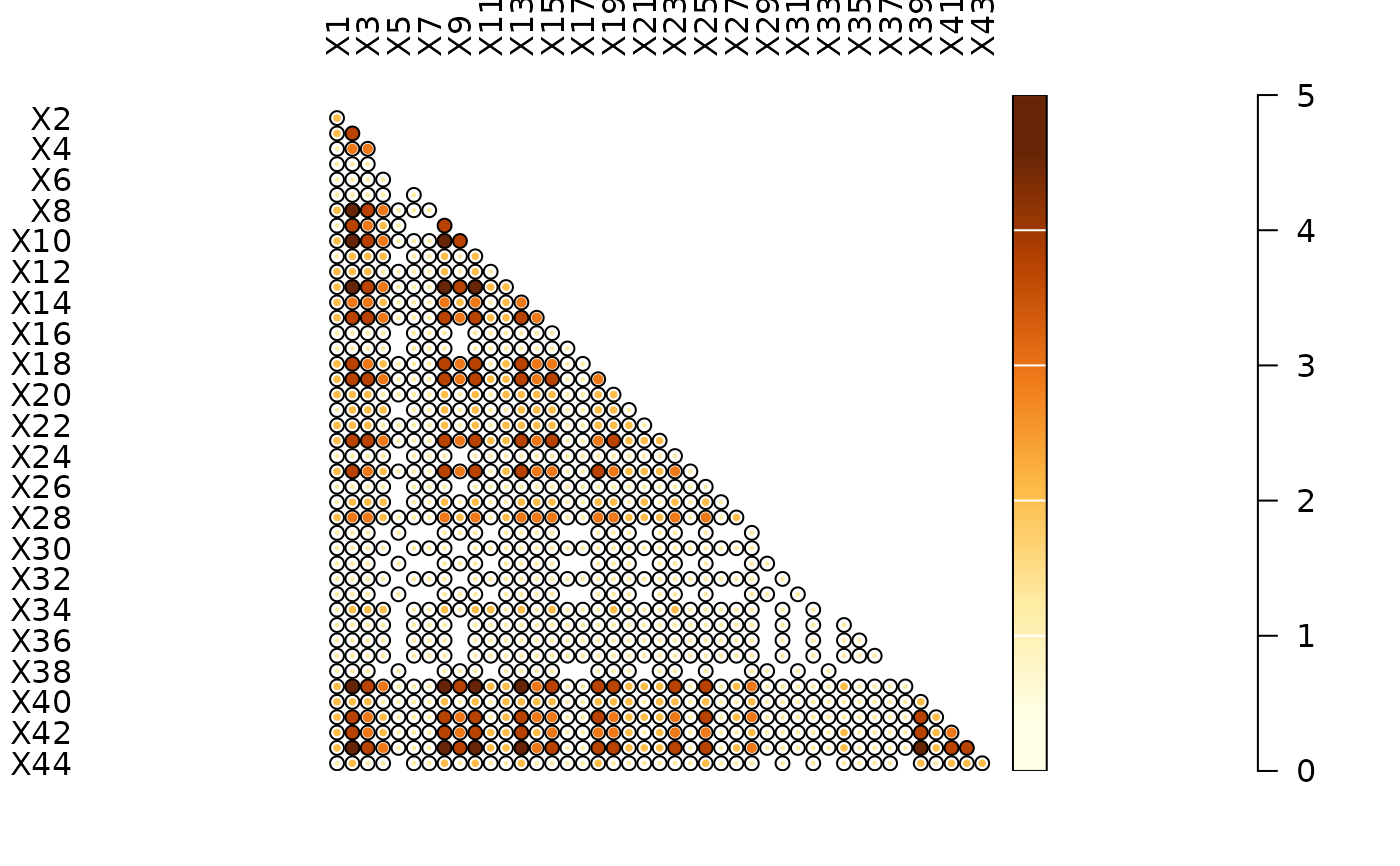Co-Occurrence

## Usage

occurrence(object, ...)

# S4 method for matrix
occurrence(object)

# S4 method for data.frame
occurrence(object)

## Arguments

object

A $$m \times p$$ numeric matrix or data.frame of count data (absolute frequencies giving the number of individuals for each category, i.e. a contingency table). A data.frame will be coerced to a numeric matrix via data.matrix().

...

Currently not used.

## Value

A stats::dist object.

## Details

A co-occurrence matrix is a symmetric matrix with zeros on its main diagonal, which works out how many times each pairs of taxa/types occur together in at least one sample.

Other diversity measures: heterogeneity(), rarefaction(), richness(), similarity(), simulate(), turnover()

N. Frerebeau

## Examples

## Data from Conkey 1980, Kintigh 1989
data("cantabria")

## Plot spot diagram of a co-occurrence matrix
occ <- occurrence(cantabria)
plot_spot(occ)